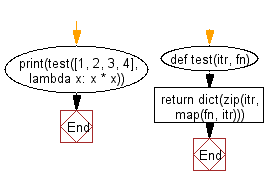﻿ Python: Map dictionary - w3resource# Python: Map dictionary

## Python dictionary: Exercise-70 with Solution

Write a Python program to map the values of a given list to a dictionary using a function, where the key-value pairs consist of the original value as the key and the result of the function as the value.

• Use map() to apply fn to each value of the list.
• Use zip() to pair original values to the values produced by fn.
• Use dict() to return an appropriate dictionary.

Sample Solution:

Python Code:

``````def test(itr, fn):
return dict(zip(itr, map(fn, itr)))
print(test([1, 2, 3, 4], lambda x: x * x))
```
```

Sample Output:

```{1: 1, 2: 4, 3: 9, 4: 16}
```

Flowchart:## Visualize Python code execution:

The following tool visualize what the computer is doing step-by-step as it executes the said program:

Python Code Editor:

Have another way to solve this solution? Contribute your code (and comments) through Disqus.

What is the difficulty level of this exercise?

Test your Programming skills with w3resource's quiz.

﻿

## Python: Tips of the Day

Decapitalizes the first letter of a string:

Example:

```def tips_decapitalize(s, upper_rest=False):
return s[:1].lower() + (s[1:].upper() if upper_rest else s[1:])
print(tips_decapitalize('PythonTips'))
print(tips_decapitalize('PythonTips', True))
```

Output:

```pythonTips
pYTHONTIPS
```